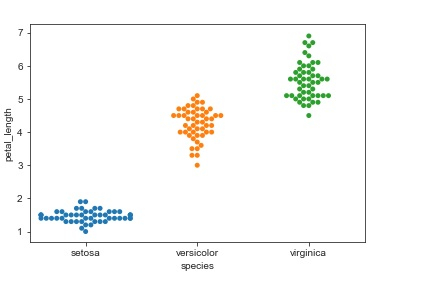# Avoid the points getting overlapped without using jitter parameter in categorical scatter plot in Python Seaborn?

We will be using Seaborn. Seaborn is a library that helps in visualizing data. It comes with customized themes and a high-level interface. This interface helps in customizing and controlling the kind of data and how it behaves when certain filters are applied to it.

The ‘stripplot’ function is used when atleast one of the variables is categorical. The data is represented in a sorted manner along one of the axes. But the disadvantage is that certain points get overlapped. This where the ‘jitter’ parameter has to be used to avoid the overlapping between variables.

It adds some random noise to the dataset, and adjusts the positions of the values along the categorical axis. But, instead of using the ‘jitter’ parameter, we can use the ‘swarmplot’ to get categorical scatter plot.

Syntax of swarmplot function

seaborn.swarmplot(x, y,data,…)

It has been demonstrated below −

## Example

import pandas as pd
import seaborn as sb
from matplotlib import pyplot as plt
sb.swarmplot(x = "species", y = "petal_length", data = my_df)
plt.show()

## Output## Explanation

• The required packages are imported.
• The input data is ‘iris_data’ which is loaded from the scikit learn library.
• This data is stored in a dataframe.
• This data is visualized using the ‘swarmplot’ function.
• Here, the dataframe is supplied as parameter.
• Also, the x and y values are specified.
• This data is displayed on the console.

Updated on: 11-Dec-2020

306 Views